# Joins in Oracle

## Joins in Oracle with Examples

In this article, I am going to discuss JOINs in Oracle with Examples. At the end of this article, you will understand what are Joins and the different categories of joins as well as how to code using ANSI and NON-ANSI format JOINs with Examples.

##### What are Joins in Oracle?

Joins in Oracle are used to retrieve data from multiple tables at a time. In relational databases, we are storing related data in multiple tables like employee details, department details, customer details, orders details, products details, etc. To combine data and retrieve data from those multiple tables then we need joins.

#### Types of Joins in Oracle:

Joins in Oracle are again classified into two types are as follows.

##### NON – ANSI format joins: (oracle 8i joins)

When we are retrieving data from multiple tables based on the “WHERE” clause condition then we called a NON-ANSI format join. NON-ANSI joins are not portable. They are again classified into three types are as follows.

1. Equi Join
2. Non-Equi Join
3. Self Join
###### The syntax for NON-ANSI joins:

SELECT * FROM TABLE NAME1, TABLE NAME2 WHERE <JOIN CONDITION>;

##### ANSI format joins: (oracle 9i joins)

When we are retrieving data from multiple tables with “on” / “using” clause conditions then we called as the join as ANSI format join. ANSI joins are portability (move from one database to another database) They are again classified as follows.

1. Inner Join
2. Outer Join (Left Outer Join, Right Outer Join, and Full Outer Join)
3. Cross Join (or) Cartesian Join
4. Natural Join
###### Syntax for ANSI joins:

SELECT * FROM <TABLE NAME1> <JOIN KEY> <TABLE NAME2 > ON <JOIN CONDITION>;

##### Examples to understand JOINs in Oracle:

We are going to use the following Course and Student tables to understand JOINs in Oracle with examples.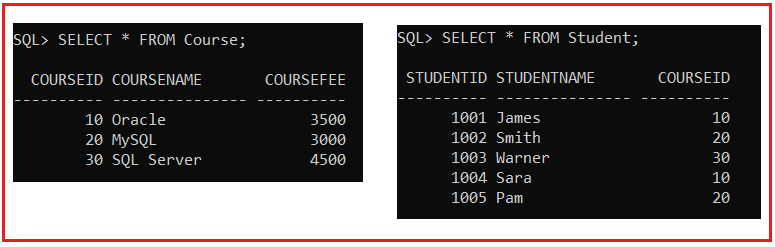Please use the below SQL script to create and populate the Course and Student tables with the required sample data.

```CREATE TABLE Course (
CourseId INT PRIMARY KEY,
CourseName VARCHAR(15),
CourseFee INT
);

INSERT INTO Course (CourseId, CourseName, CourseFee) VALUES (10, 'Oracle', 3500);
INSERT INTO Course (CourseId, CourseName, CourseFee) VALUES (20, 'MySQL', 3000);
INSERT INTO Course (CourseId, CourseName, CourseFee) VALUES (30, 'SQL Server', 4500);

CREATE TABLE Student (
StudentId INT PRIMARY KEY,
StudentName VARCHAR(15),
CourseId INT
);

INSERT INTO Student (StudentId, StudentName, CourseId) VALUES (1001, 'James', 10);
INSERT INTO Student (StudentId, StudentName, CourseId) VALUES (1002, 'Smith', 20);
INSERT INTO Student (StudentId, StudentName, CourseId) VALUES (1003, 'Warner', 30);
INSERT INTO Student (StudentId, StudentName, CourseId) VALUES (1004, 'Sara', 10);
INSERT INTO Student (StudentId, StudentName, CourseId) VALUES (1005, 'Pam', 20);
```
##### EQUI Join in Oracle:

Retrieving data from multiple tables based on “equal operator ( = ) ” is called an EQUI join. When we use EQUI join between two (or) more than two tables the common column (or) common field name is no need to be the same name (but recommend). The common column (or) common field datatype must be matched.

When we perform any join operation between tables there is no need to have a relationship(optional) (i.e. primary key & foreign key relation). EQUI join always retrieves only matching data/matching rows.

###### Syntax:

WHERE <TABLE NAME1>.<COMMON COLUMN> = <TABLE NAME2>.<COMMON COLUMN>;
(OR)
WHERE <TN1 ALIAS NAME>.<COMMON COLUMN> = <TN2 ALIAS NAME>.<COMMON COL>;

##### EQUI Join Example in Oracle:

Write a Query to retrieve student and the corresponding course details from Course and Student tables by using EQUI join?

SELECT * FROM Student, Course WHERE CourseId = CourseId;

When you execute the above query, you will get the following error i.e. ORA-00918: column ambiguously defined.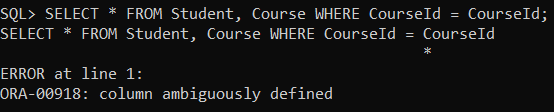Note: In the above example, we get an error i.e. “column ambiguously defined”. To overcome this error then we should use a table name as an identity to ambiguous column CourseId like below,

SELECT * FROM Student, Course WHERE Student.CourseId = Course.CourseId;
Or
SELECT * FROM Student S, Course C WHERE S.CourseId = C.CourseId;

So, when you execute the above queries, you will get the output as expected as shown in the below image.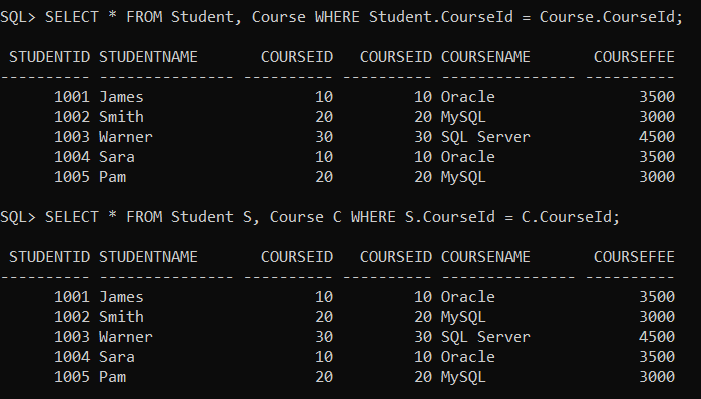##### Rule of Joining Tables in Oracle:

A row in the first table is compared with all rows of the second table.

###### Example:

Write a Query to retrieve student, course details from tables if CourseId is 20.

SELECT * FROM Student S, Course C WHERE S.CourseId = C.CourseId AND C.CourseId = 20;

When you execute the above query, you will get the following output.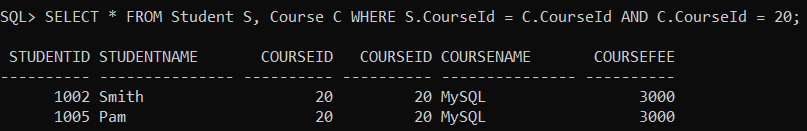##### In ANSI Format: INNER JOIN / EQUI JOIN:

Inner join in Oracle is similar to EQUI join i.e. retrieving data from multiple tables with the “ON” clause condition.

###### Syntax:

ON <TABLE NAME1>.<COMMON COLUMN> = <TABLE NAME2>.<COMMON COLUMN>;
(OR)
ON <TN1 ALIAS NAME>.<COMMON COLUMN> = <TN2 ALIAS NAME>.<COMMON COLUMN>;

###### Example:

Write a Query to retrieve student, course details from Course and Student tables by using INNER JOIN.

SELECT * FROM Student INNER JOIN Course ON Student.CourseId = Course.CourseId;

When you execute the above query, you will get the following output.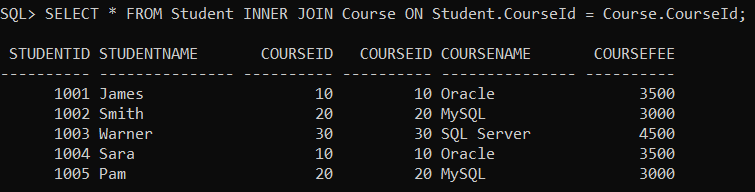##### WHY DID ANSI JOIN?

These joins are introduced in Oracle 9i. The main advantage of ANSI joins is portability. It means that we can move to join statements from one database to another database without making any changes as it is the join statements are executed in other databases.

##### Outer joins in oracle:

In the above EQUI / Inner Join we are retrieving only matching rows but not unmatching rows from multiple tables. So, to overcome this problem we need to use the “OUTER JOINS” mechanism. These are again three types:

1. Left outer join
2. Right outer join
3. Full outer join
##### LEFT OUTER JOIN in Oracle:

Retrieving all rows (matching & unmatching) from the left side table. But retrieving matching rows from the right side table.

###### ANSI format:

SELECT * FROM Student S LEFT OUTER JOIN Course C ON S.CourseId =C.CourseId;

###### NON – ANSI format:

When we write outer joins in NON-ANSI format then we should use the Join operator (+).

SELECT * FROM Student S, Course C WHERE S.CourseId =C.CourseId(+);

When you execute the above queries, you will get the following output.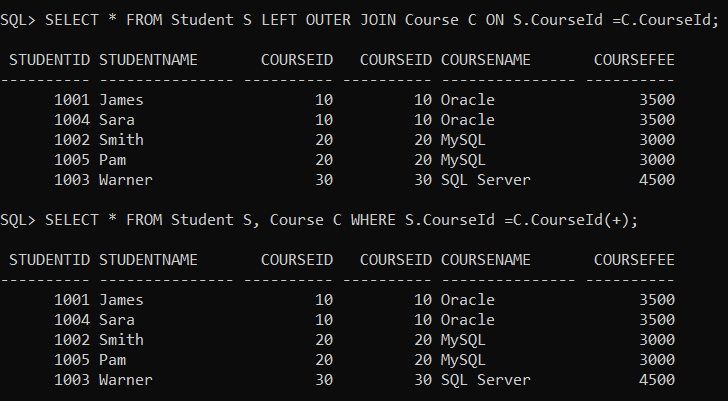##### RIGHT OUTER JOIN in Oracle:

Retrieving all rows (matching & unmatching) from the right side table but retrieving only the matching rows from the left side table.

###### ANSI FORMAT:

SELECT * FROM Student S RIGHT OUTER JOIN Course C ON S.CourseId =C.CourseId;

###### NON-ANSI FORMAT:

SELECT * FROM Student S, Course C WHERE S.CourseId(+) =C.CourseId;

When you execute the above queries, you will get the following output.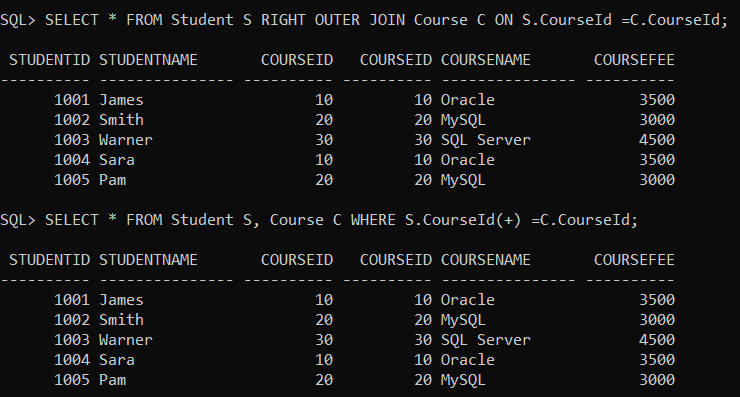##### FULL OUTER JOIN in Oracle:

Retrieving matching and also unmatching rows from both sides tables.

###### ANIS FORMAT:

SELECT * FROM Student S FULL OUTER JOIN Course C ON S.CourseId =C.CourseId;

When you execute the above query, you will get the following output.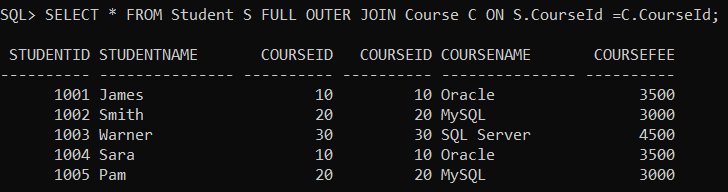###### NON – ANSI FORMAT:

SELECT * FROM Student S, Course C WHERE S.CourseId(+) =C.CourseId(+);

When you try to execute the above query, you will get the following error.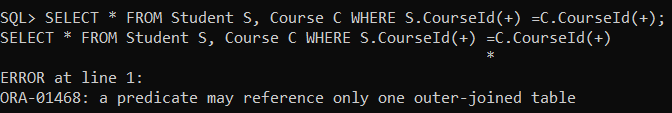Note: NON-ANSI format is not supporting the full outer join mechanism. So when we want to implement full outer join in NON -ANSI format then we combined the results of left outer and right outer joins by using the “UNION” Operator.

###### Example:

SELECT * FROM Student S, Course C WHERE S.CourseId =C.CourseId(+)
UNION
SELECT * FROM Student S, Course C WHERE S.CourseId(+) =C.CourseId;

When you execute the above, you will get the output as expected as shown in the below image.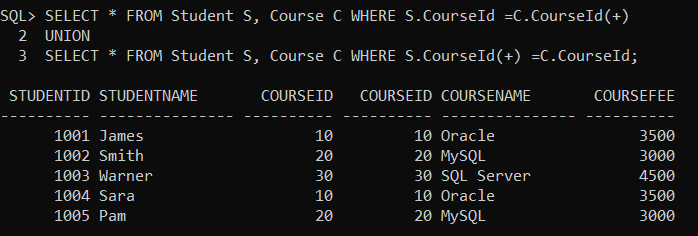##### NON-EQUI JOIN in Oracle:

Retrieving data from multiple tables based on any condition except equal operator condition is called a NON-EQUI join. In the join, we can use the operators such as <,>,<=,>=, and, between,………etc.

###### Example: NON-ANSI:

SELECT * FROM Student S, Course C WHERE S.CourseId > C.CourseId;

###### Example: ANSI:

SELECT * FROM Student S JOIN Course C ON S.CourseId > C.CourseId;

When you execute the above queries, you will get the following output.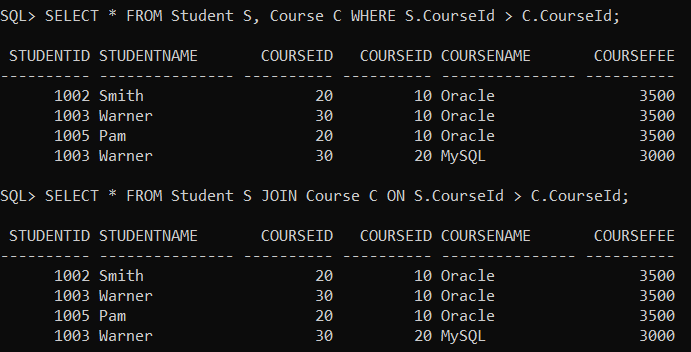##### CROSS JOIN / CARTISEAN JOIN in Oracle:

Joining two (or) more than two tables without any condition is called a “cross / Cartesian join”. In cross join, each row of the first table will join joins with each row of the second table. That means a first table is having “m” no. of rows and a second table is having “n” no. of rows then the result is m x n no. of rows.

###### Example: ANSI Format

SELECT * FROM STUDENT CROSS JOIN COURSE;

When you execute the above query, you will get the following output.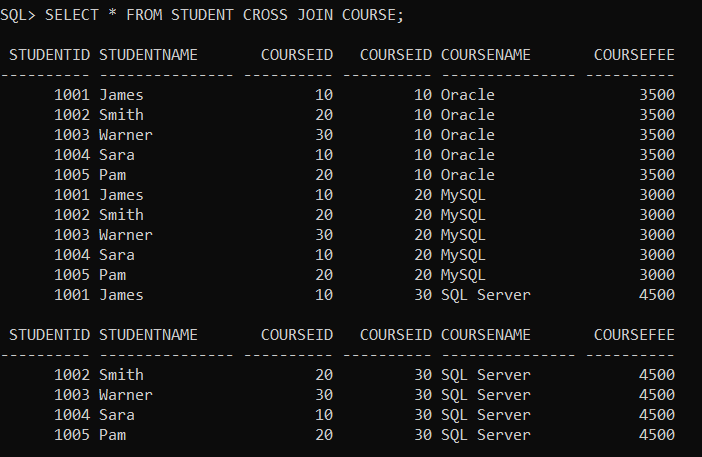###### Example: NON-ANSI Format

SELECT * FROM STUDENT, COURSE;

When you execute the above queries, you will get the same output as the previous one.

##### NATURAL JOIN in Oracle:

Natural join is similar to Equi join. When we use natural join, we should have a common column name. This column datatype must be matched. Whenever we are using natural join there is no need to write a joining condition explicitly because internally oracle server is preparing the joining condition based on an “equal operator(=)” with column name automatically. By using natural join, we avoid duplicate columns while retrieving data from multiple tables.

###### Example:

SELECT * FROM STUDENT S NATURAL JOIN COURSE C;

When you execute the above query, you will get the following output.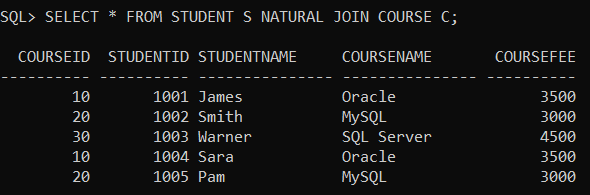##### USING CLAUSE in Oracle:

In ANSI format joins whenever we join two or more than two tables, then instead of the “ON” clause, we can use the “USING” clause also. It returns the common column only one time.

###### Example:

SELECT * FROM STUDENT S INNER JOIN COURSE C USING(S.CourseId);

When you execute the above query, you will get the following output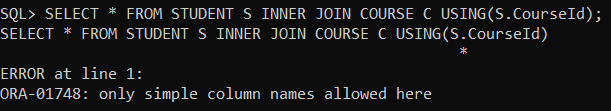Note: When we use the “USING” clause with a common column name there is no need to prefix with a table alias name.

Example:
USING(S.CourseId);——-ERROR
USING(CourseId);——ALLOWED

###### Example:

SELECT * FROM STUDENT S INNER JOIN COURSE C USING(CourseId);

When you execute the above query, you will get the following output.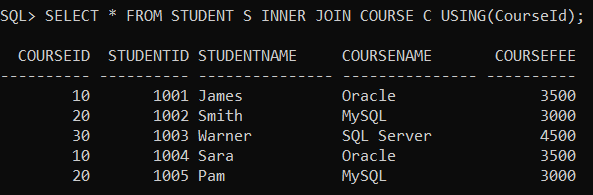Note: In this article, I just gave an overview of Joins in Oracle. From the next articles onwards, I am going to discuss Joins in Oracle with real-time examples using both ANSI and NON-ANSI formats.

In the next article, I am going to discuss INNER JOIN in Oracle with Real-time Examples. Here, in this article, I try to explain JOINs in Oracle with Examples and I hope you enjoy this article.